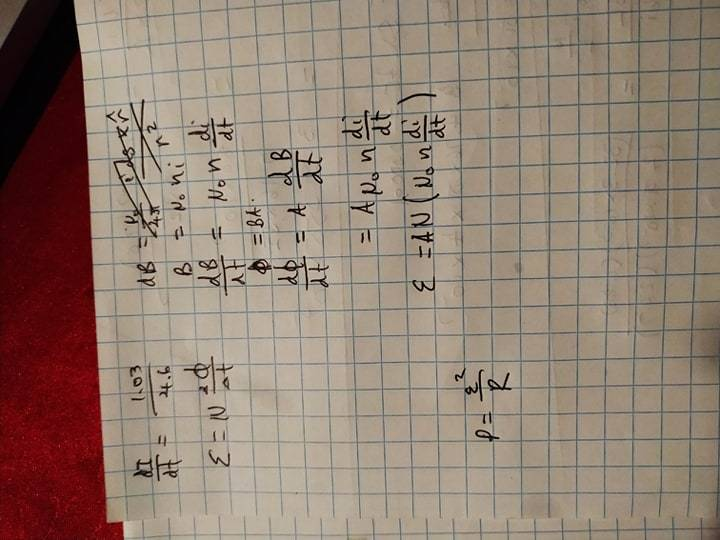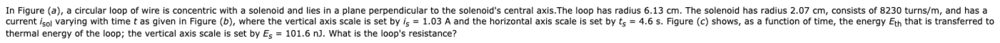# Circular loop of wire is concentric with a solenoid

Homework Statement:
Find the loops resistance
Relevant Equations:
N/A
Question:
In Figure (a), a circular loop of wire is concentric with a solenoid and lies in a plane perpendicular to the solenoid's central axis.The loop has radius 6.13 cm. The solenoid has radius 2.07 cm, consists of 8230 turns/m, and has a current i_sol varying with time t as given in Figure (b), where the vertical axis scale is set by i_s = 1.03 A and the horizontal axis scale is set by ts = 4.6 s. Figure (c) shows, as a function of time, the energy E that is transferred to thermal energy of the loop; the vertical axis scale is set by Es = 101.6 nJ. What is the loop's resistance?

I want to find B, the magnetic flux density such that I can work out what the EMF is.
From the EMF I can use
$$P = \frac{EMF^2}{R}$$

To work out R as I know what P is since that is J/s.

However how can I find B when:
$$B = \frac{\mu N I}{2R}$$

and I don't know what the current (I) is? As the current varies over time.

If someone could provide what is going on in terms of the physics and the required next steps that would be great!

#### Attachments

•pict_30_28.gif
4 KB · Views: 182
•Delta2

Delta2
Homework Helper
Gold Member
Look carefully at figures (b) and c. From (b) you can determine completely the function I(t) (that is the current as a function of time t) and from (c) you can determine completely the function E(t).

Look carefully at figures (b) and c. From (b) you can determine completely the function I(t) (that is the current as a function of time t) and from (c) you can determine completely the function E(t).
Sorry I don't follow, I can find the rate of change of these with regards to time, however I don't see anything I can do it with

Delta2
Homework Helper
Gold Member
Sorry I don't follow, I can find the rate of change of these with regards to time, however I don't see anything I can do it with
How can you find the rate of change of I and E w.r.t to time t?

And of course you can do many things with it, from the rate of change of current(which will be the same (up to some multiplicative constants) to rate of change of magnetic field B of the solenoid) and Faraday's law of induction you can calculate the EMF through the circular wire loop. From the rate of change of energy you can calculate the Power, due to the EMF and current, that is dissipate as heat in the resistance R of the loop.

How can you find the rate of change of I and E w.r.t to time t?

And of course you can do many things with it, from the rate of change of current(which will be the same (up to some multiplicative constants) to rate of change of magnetic field B of the solenoid) and Faraday's law of induction you can calculate the EMF through the circular wire loop. From the rate of change of energy you can calculate the Power, due to the EMF and current, that is dissipate as heat in the resistance R of the loop.
To confirm, is this what you meant? I have played with the equations a little. Also, n is the density of coils/mHowever I am not provided with the length of the solenoid?

Delta2
Homework Helper
Gold Member
Your equations appear to be correct, but it all depends what A and N (the capital N in the last equation for emf) are. So please tell me what is A and what is N

And you don't need to know the length of the solenoid to answer the question. It seems to be that the solenoid is supposed to have very large length, theoretically infinite.

Your equations appear to be correct, but it all depends what A and N (the capital N in the last equation for emf) are. So please tell me what is A and what is N

And you don't need to know the length of the solenoid to answer the question. It seems to be that the solenoid is supposed to have very large length, theoretically infinite.Judging by this, A = pi * 0.0207^2 (Solenoids radius right?) And n (Not N) = 8230/m

Delta2
Homework Helper
Gold Member
Well you are correct for A (and for n). But tell me why you chose A to be the cross section area of the solenoid and not the cross section area of the wire loop

And also tell me what is N

Well you are correct for A (and for n). But tell me why you chose A to be the cross section area of the solenoid and not the cross section area of the wire loop

And also tell me what is N
Is N = 1? Because we have only a single ring? And I chose A to be the cross section of the area because that's what the equation for B is. The magnetic flux density through the solenoid as it's within the ring, but we treat the ring like a solenoid, so N = 1.

Delta2
Homework Helper
Gold Member
Is N = 1? Because we have only a single ring? And I chose A to be the cross section of the area because that's what the equation for B is. The magnetic flux density through the solenoid as it's within the ring, but we treat the ring like a solenoid, so N = 1.
yes you are correct N=1. So I guess all you have to do is plug in the numbers to the equations. You also can find ##P=\frac{dE}{dt}## from figure (c), so you can solve for the resistance of the loop.

yes you are correct N=1. So I guess all you have to do is plug in the numbers to the equations. You also can find ##P=\frac{dE}{dt}## from figure (c), so you can solve for the resistance of the loop.
I got 0.00054996 Ohms (Which I hope is correct??). Thank you so much!

•Delta2